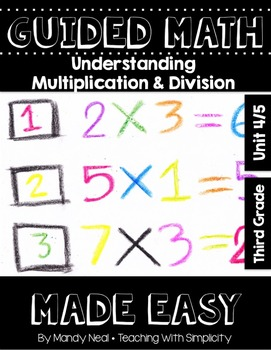# Third Grade Guided Math ~ Understanding Multiplication and DivisionSubject
Resource Type
Format
Zip (32 MB|115 pages)
Standards
\$8.50
\$8.50

### Description

Third grade guided math and math workshop activities.

Yes, guided math can be made easy! It can be made easy, especially if you are using the Guided Math Made Easy Series!

This Understanding Multiplication and Division unit is one of 14 units that will be a part of the Guided Math Made Easy Series for Third Grade.

Each unit contains the same components. The consistency of the components will allow students, as well as the teacher, to become familiar with the routines.

Each lesson contains the following components:

Objectives

Essential questions

Important vocabulary

Necessary materials

Anchor chart example

Whole group lesson

Three levels of instruction (Approaching Level, On-Level, and Advanced)

Pre and Post Assessment

Problem of the Day

Number of the Day activities

Work Mats

Independent interactive notebook practice

Flip-A-Card Math Stations

For an in-depth look, be sure to check out the preview!!

If you have any questions, please email me at mandy@teachingwithsimplicity.com!

Total Pages
115 pages
Included
Teaching Duration
2 Weeks
Report this Resource to TpT
Reported resources will be reviewed by our team. Report this resource to let us know if this resource violates TpT’s content guidelines.

### Standards

to see state-specific standards (only available in the US).
Fluently multiply and divide within 100, using strategies such as the relationship between multiplication and division (e.g., knowing that 8 × 5 = 40, one knows 40 ÷ 5 = 8) or properties of operations. By the end of Grade 3, know from memory all products of two one-digit numbers.
Use multiplication and division within 100 to solve word problems in situations involving equal groups, arrays, and measurement quantities, e.g., by using drawings and equations with a symbol for the unknown number to represent the problem.
Interpret whole-number quotients of whole numbers, e.g., interpret 56 ÷ 8 as the number of objects in each share when 56 objects are partitioned equally into 8 shares, or as a number of shares when 56 objects are partitioned into equal shares of 8 objects each. For example, describe a context in which a number of shares or a number of groups can be expressed as 56 ÷ 8.